# Math 1110- MAPLE LAB 1

Maple Lab I

## Math 111 Lab Number 1 - Introduction to Maple with Matrices

 Math 111 - Introduction to Linear Algebra - Lab 1

The purpose of this lab is to introduce you to a computer program called Maple and begin to use it in our study of matrices. Maple is mathematical software which can do some fairly advanced graphing and computational work in order to investigate a broad range of topics. We will mainly be using its capabilities with matrices, since this is so useful in the study of linear algebra. Maple is currently located on the computers in Engineering 136 and Engineering 138.

After booting up, Maple will display a blank worksheet, with the words "File," "Edit," "View," "Insert," "Format," "Options," "Window," and "Help" across the top of the screen. These are pull down menus which are accessed by positioning the arrow on the menu heading and pushing down on the mouse button. Once the menu appears, select the desired command by sliding the arrow down and releasing the mouse button.

Now let's begin by entering a simple matrix. At the prompt (the thing that looks like part of an arrow) type the following then hit enter (you should always hit enter when you complete a line):

> with(linalg):

This activates a package containing many functions specific to linear algebra topics. Note that after typing this line the computer will warn you there are a few new definitions. Don't worry about this. You must type the with(linalg): command at the start of every one of your computer labs or else the computer won't know what you're talking about. At the next prompt type:

> A:=matrix(2,3,[4,-1,6,5,3,2]);You have just entered your first matrix into Maple. Note that the first line is what you type then Maple will show you the matrix. So, what did the line you typed mean? That's simple. We started with a capital letter- that's the name we want to give the matrix. Then you typed ":=" that tells the computer that what you put before the ":=" was the name and what is after the ":=" is the object you want to name. Next we typed "matrix" that tells the computer we're building a matrix. Then after the parentheses, we typed the size of the matrix (2 rows by 3 columns). Inside the square brackets we list the entries of the matrix by going across the rows. After closing our brackets and parentheses we typed a semicolon. You must always use a semicolon at the end of a line to tell Maple that you've completed the command.

Here's another example to try. Note here that I am naming my matrix "B."

> B:=matrix(3,4,[2,3,6,7,-1,-5,8,14,3,3,4,0]);Note that you cannot name a matrix with the name "D" or "E"- Maple has other uses for these letters.

Gauss Jordan Elimination

Now that we know how to enter a matrix let's see what Maple can do with them. Say you want to solve the following system of equations:
x+y-z=6
2x-y+z=-9
x-2y+3z=1

We learned at the beginning of the semester that if we put the coefficients of these equations into a matrix and then perform Gauss-Jordan elimination that will give us the solution we are looking for. So I need to put the coefficients of the equations into a matrix, call the matrix, C.

> C:=matrix(3,4,[1,1,-1,6,2,-1,1,-9,1,-2,3,1]);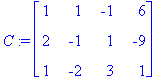To put this matrix in Gauss-Jordan form we use the command rref. The letters stand for Row Reduce Echelon Form.

> rref(C);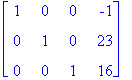Thus, the solution to the system of equations is (-1,23,16). It was a lot less work to plug this into the computer then to do it by hand.

Matrix Arithmetic

Maple can also perform matrix arithmetic for us. As we know, two matrices can be added or subtracted. The first thing I want to do is find the sum of B and C. (These matrices were defined earlier in the lab and Maple will keep "B" and "C' to mean the matrices defined above until we log off or until we define something else using the same letters.) So to compute B+C, type:To multiply a matrix by a scalar type (i.e. to find 4 times B):

> scalarmul(B,4);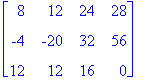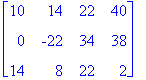So, since subtracting C from B is the same as adding -1 times C to B, in order to find B-C we use (1)B+(-1)C: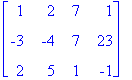Matrix Multiplication

The next thing that would be handy to know how to do on Maple would be to know how to multiply matrices. Before we start, remember that the sizes of two matrices must correspond in order for us to multiply them. (i.e. The number of columns of the first must match the number of rows in the second matrix.)

So, let's define two matrices:

> F:=matrix(4,3,[1,2,3,4,5,6,7,8,9,1,2,3]);> G:=matrix(3,2,[0,18,-2,44,1,9]);Then, to compute FG, use the command:

> multiply(F,G);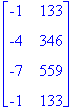Matrix Inverses

The last matrix operation we want to learn how to do on Maple is to find the inverse of a matrix. Again, we start by defining a matrix. Remember that in order to have an inverse, a matrix must be square.

> H:=matrix(4,4,[2,9,6,5,-1,-2,3,8,14,-7,8,8,0,4,2,1]);To find the inverse, simply use the following command:

> inverse(H);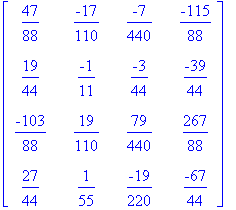Here's a couple more hints before you start the homework for this lab:

1) Remember that the end of each command has a semicolon- that's your way of telling the computer that you have completed the command. You must enter the semicolon for the computer to understand you.

2) Do not save your work to the hard drive. It is erased every 24 hours. If you need to save your work, save it to a disk. The save command is located under the file menu.

3) When you finish the exercises below, you will need to print them (and only them- don't print any of the work you've done above. To start with a new Maple worksheet you can use the "New" command under the "File" menu.) Then to print, use the print command which is also located under the "File" menu.

4) When you are completely done with the lab you need to go to the File menu and select quit. This will take you back to the Windows screen. Once you arrive there, you need to log off by putting your arrow on the start button in the lower left corner and selecting "log off math user."

Exercises:

Define the following matrices and perform the indicated operations:

>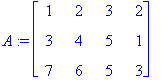>>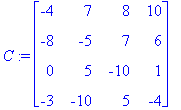1. Find 3A+6B.
2. Find 2B-A.
3. Find BC.
4. Find the inverse of C.
5. Solve the following system of linear equations:
x+4y+6z-w=14
2x+5y+2w=20
-3x+z+4w=16
7x-3y+4z+2w=2
6. Solve the following system of linear equations:
4x-2y-3z=-23
-4x+3y+z=11
8x-5y+4z=6